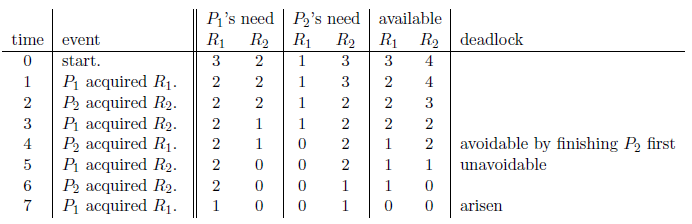Time Limit : sec, Memory Limit : KB
English

You are working on an analysis of a system with multiple processes and some kinds of resource (such as memory pages, DMA channels, and I/O ports). Each kind of resource has a certain number of instances. A process has to acquire resource instances for its execution. The number of required instances of a resource kind depends on a process. A process finishes its execution and terminates eventually after all the resource in need are acquired. These resource instances are released then so that other processes can use them. No process releases instances before its termination. Processes keep trying to acquire resource instances in need, one by one, without taking account of behavior of other processes. Since processes run independently of others, they may sometimes become unable to finish their execution because of deadlock.

A process has to wait when no more resource instances in need are available until some other processes release ones on their termination. Deadlock is a situation in which two or more processes wait for termination of each other, and, regrettably, forever. This happens with the following scenario: One process A acquires the sole instance of resource X, and another process B acquires the sole instance of another resource Y; after that, A tries to acquire an instance of Y, and B tries to acquire an instance of X. As there are no instances of Y other than one acquired by B, A will never acquire Y before B finishes its execution, while B will never acquire X before A finishes. There may be more complicated deadlock situations involving three or more processes.

Your task is, receiving the system's resource allocation time log (from the system's start to a certain time), to determine when the system fell into a deadlock-unavoidable state. Deadlock may usually be avoided by an appropriate allocation order, but deadlock-unavoidable states are those in which some resource allocation has already been made and no allocation order from then on can ever avoid deadlock.

Let us consider an example corresponding to Sample Input 1 below. The system has two kinds of resource $R_1$ and $R_2$, and two processes $P_1$ and $P_2$. The system has three instances of $R_1$ and four instances of $R_2$. Process $P_1$ needs three instances of $R_1$ and two instances of $R_2$ to finish its execution, while process $P_2$ needs a single instance of $R_1$ and three instances of $R_2$. The resource allocation time log is given as follows.At time 4, $P_2$ acquired $R_1$ and the number of available instances of $R_1$ became less than $P_1$'s need of $R_1$. Therefore, it became necessary for $P_1$ to wait $P_2$ to terminate and release the instance. However, at time 5, $P_1$ acquired $R_2$ necessary for $P_2$ to finish its execution, and thus it became necessary also for $P_2$ to wait $P_1$; the deadlock became unavoidable at this time.

Note that the deadlock was still avoidable at time 4 by finishing $P_2$ first (Sample Input 2).

Input

The input consists of a single test case formatted as follows.

$p$ $r$ $t$
$l_1$ ... $l_r$
$n_{1,1}$ ... $n_{1,r}$
...
$n_{p,1}$ ... $n_{p,r}$
$P_1$ $R_1$
...
$P_t$ $R_t$

$p$ is the number of processes, and is an integer between 2 and 300, inclusive. The processes are numbered 1 through $p$. $r$ is the number of resource kinds, and is an integer between 1 and 300, inclusive. The resource kinds are numbered 1 through $r$. $t$ is the length of the time log, and is an integer between 1 and 200,000, inclusive. $l_j$ $(1 \leq j \leq r)$ is the number of initially available instances of the resource kind $j$, and is an integer between 1 and 100, inclusive. $n_{i,j}$ $(1 \leq i \leq p, 1 \leq j \leq r)$ is the number of resource instances of the resource kind $j$ that the process $i$ needs, and is an integer between 0 and $l_j$ , inclusive. For every $i$, at least one of $n_{i,j}$ is non-zero. Each pair of $P_k$ and $R_k$ $(1 \leq k \leq t)$ is a resource allocation log at time $k$ meaning that process $P_k$ acquired an instance of resource $R_k$.

You may assume that the time log is consistent: no process acquires unnecessary resource instances; no process acquires instances after its termination; and a process does not acquire any instance of a resource kind when no instance is available.

Output

Print the time when the system fell into a deadlock-unavoidable state. If the system could still avoid deadlock at time $t$, print -1.

2 2 7
3 4
3 2
1 3
1 1
2 2
1 2
2 1
1 2
2 2
1 1

5

2 2 5
3 4
3 2
1 3
1 1
2 2
1 2
2 1
2 2

-1